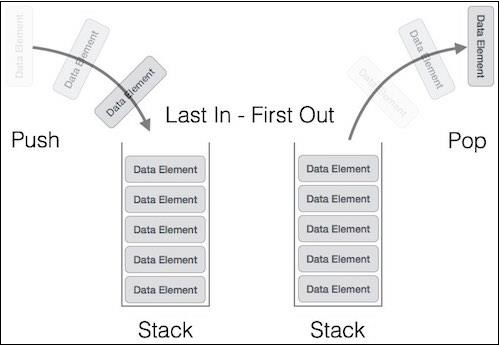# Stack Data Structure in Javascript

JavascriptWeb DevelopmentFront End Technology

A stack is an Abstract Data Type (ADT), commonly used in most programming languages. It is named stack as it behaves like a real-world stack, for example – a deck of cards or a pile of plates, etc.A stack allows operations at one end only. This feature makes it LIFO data structure. LIFO stands for Last-in-first-out. Here, the element which is placed (inserted or added) last, is accessed first. In stack terminology, insertion operation is called PUSH operation and removal operation is called POP operation.

The following diagram shows the operations on the stack −Following is the complete Javascript class to represent a Stack −

## Example

class Stack {
constructor(maxSize) { // Set default max size if not provided
if (isNaN(maxSize)) {
maxSize = 10;
}
this.maxSize = maxSize; // Init an array that'll contain the stack values.
this.container = [];
}
display() {
console.log(this.container);
}
isEmpty() {
return this.container.length === 0;
}
isFull() {
return this.container.length >= this.maxSize;
}
push(element) { // Check if stack is full
if (this.isFull()) {
console.log("Stack Overflow!") return;
}
this.container.push(element)
}
pop() { // Check if empty
if (this.isEmpty()) {
console.log("Stack Underflow!") return;
}
this.container.pop()
}
peek() {
if (isEmpty()) {
console.log("Stack Underflow!");
return;
}
return this.container[this.container.length - 1];
}
clear() {
this.container = [];
}
}